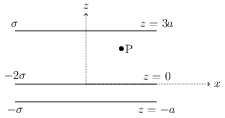# Electric Field due to Uniformly Charged Infinite Plane Sheet

## Problems from IIT JEE

Problem (IIT JEE 2005):Three infinitely long charge sheets are placed parallel to $x\text{-}y$ plane as shown in the figure. The electric field at point P is,

1. $\frac{2\sigma}{\epsilon_0}\hat{k}$
2. $-\frac{2\sigma}{\epsilon_0}\hat{k}$
3. $\frac{4\sigma}{\epsilon_0}\hat{k}$
4. $-\frac{4\sigma}{\epsilon_0}\hat{k}$

Solution: The electric field at P is given by, \begin{align} \vec{E}=\frac{-\sigma}{2\epsilon_0} \hat{k}+\frac{-2\sigma}{2\epsilon_0}\hat{k}+\frac{\sigma}{2\epsilon_0} (-\hat{k})=-\frac{2\sigma}{\epsilon_0}\hat{k}.\nonumber \end{align}